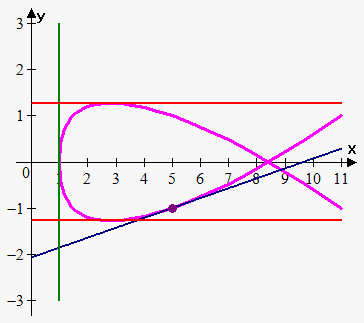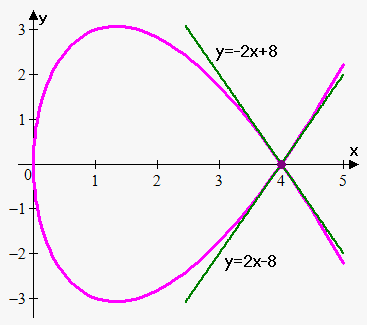# Tangent Line to Parametric Curves

Sometimes function is defined parametrically, but we still need to find equation of tangent line.

So, let parametric curve is defined by equations $x=f{{\left({t}\right)}}$ and $y=g{{\left({t}\right)}}$.

Suppose $f$ and $g$ are differentiable functions and we want to find the tangent line at a point on the curve where $y$ is also a differentiable function of $x$.

Then using Chain Rule we can write $\frac{{{d}{y}}}{{{d}{t}}}=\frac{{{d}{y}}}{{{d}{x}}}\frac{{{d}{x}}}{{{d}{t}}}$.

If $\frac{{{d}{x}}}{{{d}{t}}}\ne{0}$ then we solve for $\frac{{{d}{y}}}{{{d}{x}}}$:

${\color{red}{{\frac{{{d}{y}}}{{{d}{x}}}=\frac{{\frac{{{d}{y}}}{{{d}{t}}}}}{{\frac{{{d}{x}}}{{{d}{t}}}}}}}}$.

This equation allows us to find the slope $\frac{{{d}{y}}}{{{d}{x}}}$ of tangent line without having to eliminate parameter ${t}$.

We see from equation that the curve has

• horizontal tangent when $\frac{{{d}{y}}}{{{d}{t}}}={0}$ (provided that $\frac{{{d}{x}}}{{{d}{t}}}\ne{0}$).
• vertical tangent when $\frac{{{d}{x}}}{{{d}{t}}}={0}$ (provided that $\frac{{{d}{y}}}{{{d}{t}}}\ne{0}$).

Example 1. Find equation of tangent line at point (5,-1) to the curve ${x}={3}{{t}}^{{4}}+{{t}}^{{2}}+{1}$ and ${y}={{t}}^{{5}}-{2}{t}$. Where curve has horizontal and vertical tangents?

$\frac{{{d}{x}}}{{{d}{t}}}={3}\cdot{4}{{t}}^{{{4}-{1}}}+{2}\cdot{{t}}^{{{2}-{1}}}={12}{{t}}^{{3}}+{2}{t}$.

$\frac{{{d}{y}}}{{{d}{t}}}={5}\cdot{{t}}^{{{5}-{1}}}-{2}\cdot{1}{{t}}^{{{1}-{1}}}={5}{{t}}^{{4}}-{2}$.

At the point with parameter value t, the slope is

$\frac{{{d}{y}}}{{{d}{x}}}=\frac{{\frac{{{d}{y}}}{{{d}{t}}}}}{{\frac{{{d}{x}}}{{{d}{t}}}}}=\frac{{{5}{{t}}^{{4}}-{2}}}{{{12}{{t}}^{{3}}+{2}{t}}}$.

Point (5,-1) corresponds to ${t}={1}$, so $\frac{{{d}{y}}}{{{d}{x}}}{\mid}_{{{t}={1}}}=\frac{{{5}\cdot{{1}}^{{4}}-{2}}}{{{12}\cdot{{1}}^{{3}}+{2}\cdot{1}}}=\frac{{3}}{{14}}$.Therefore, equation of tangent line is ${y}+{1}=\frac{{3}}{{14}}{\left({x}-{5}\right)}$ or ${y}=\frac{{3}}{{14}}{x}-\frac{{29}}{{14}}$ (blue line).

Curve has horizontal tangent when $\frac{{{d}{y}}}{{{d}{t}}}={0}$ or ${5}{{t}}^{{4}}-{2}={0}$. So, tangent line is horizontal at ${t}=\pm{\sqrt[{{4}}]{{\frac{{2}}{{5}}}}}\approx\pm{0.795271}$.

When ${t}={0.795271}$ ${\left({x},{y}\right)}={\left({1.8324576},-{1.272433}\right)}$.

When ${t}=-{0.795271}$ ${\left({x},{y}\right)}={\left({1.8324576},{1.272433}\right)}$.

Curve has vertical tangent when $\frac{{{d}{x}}}{{{d}{t}}}={0}$ or ${12}{{t}}^{{3}}+{2}{t}={0}$. So, tangent line is horizontal at ${t}={0}$.

When ${t}={0}$ ${\left({x},{y}\right)}={\left({0},{0}\right)}$.

As appears from graph our answers are correct (green line is vertical tangent, red lines are horizontal tangents).

Finally, let's see example when there can be more than one tangent line at point.

Example 2. Find equation of tangent line to ${x}={{t}}^{{2}}$, ${y}={{t}}^{{3}}-{4}{t}$ at point ${\left({4},{0}\right)}$.

First let's find values of ${t}$ for which ${x}={4}$ and ${y}={0}$.

So, ${{t}}^{{2}}={4}$ when ${t}=\pm{2}$ and ${{t}}^{{3}}-{4}{t}={t}{\left({{t}}^{{2}}-{4}\right)}={0}$ when ${t}={0}$ and ${t}=\pm{2}$.So, ${x}={4}$, ${y}={0}$ when ${t}=\pm{2}$. Note that we obtained two values for ${t}$, so there will be two tangent lines.

Now, let's find derivative:

$\frac{{{d}{y}}}{{{d}{x}}}=\frac{{\frac{{{d}{y}}}{{{d}{t}}}}}{{\frac{{{d}{x}}}{{{d}{t}}}}}=\frac{{{3}{{t}}^{{2}}-{4}}}{{{2}{t}}}$.

When ${t}={2}$ we have that $\frac{{{d}{y}}}{{{d}{x}}}{\mid}_{{{t}={2}}}=\frac{{{3}\cdot{{2}}^{{2}}-{4}}}{{{2}\cdot{2}}}={2}$.

So, first tangent line is ${y}-{0}={2}{\left({x}-{4}\right)}$ or ${y}={2}{x}-{8}$.

When ${t}=-{2}$ we have that $\frac{{{d}{y}}}{{{d}{x}}}-{\mid}_{{{t}={2}}}=\frac{{{3}\cdot{{\left(-{2}\right)}}^{{2}}-{4}}}{{{2}\cdot{\left(-{2}\right)}}}=-{2}$.

Thus, second tangent line is ${y}-{0}=-{2}{\left({x}-{4}\right)}$ or ${y}=-{2}{x}+{8}$.

As can be seen there are two tangents at point where curve crosses itself, so there are one tangent line for each instance that the curve goes through the point.